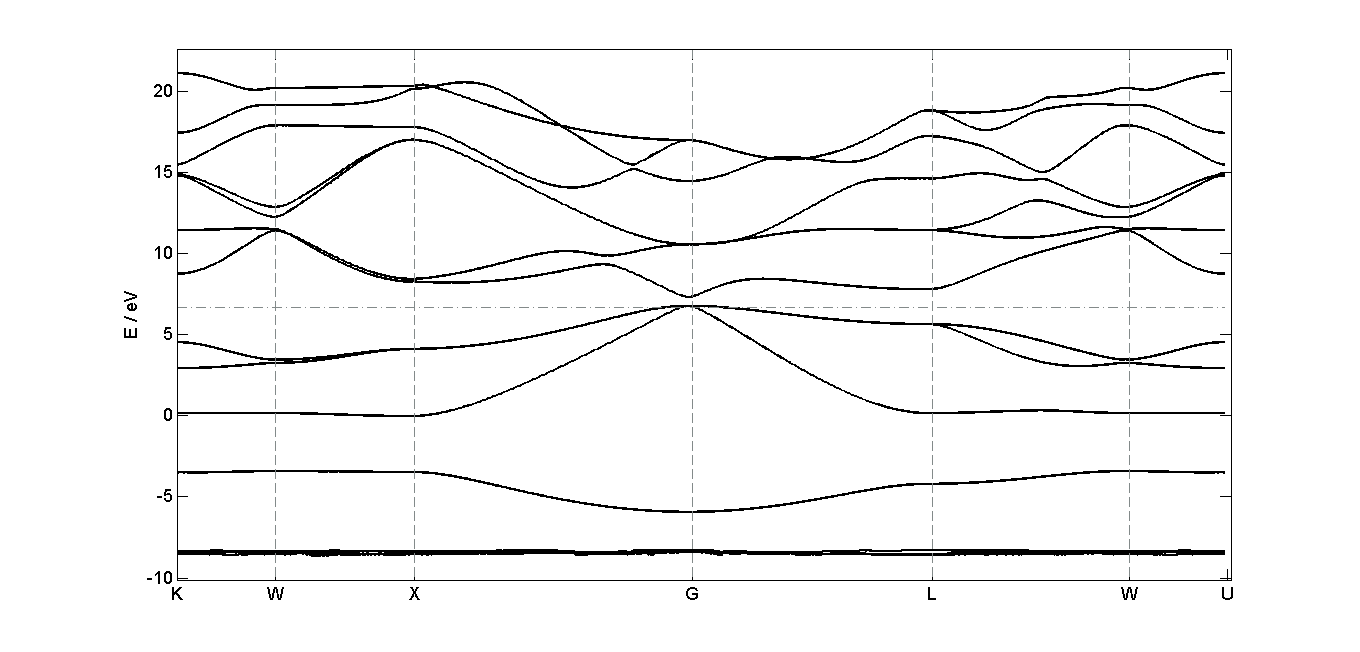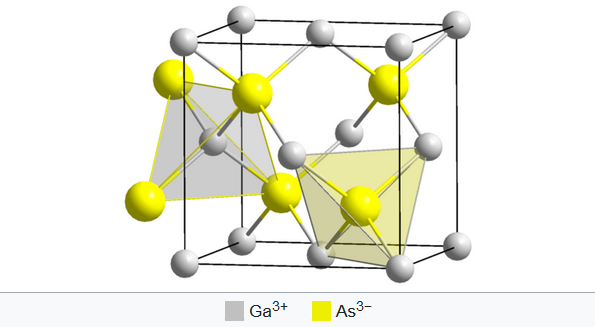## Bandstructure of gallium arsenide (GaAs)

The electron bandstructure for gallium arsenide (zincblende structure) was calculated using the program Quantum Espresso (version 5.3.0). GaAs is a compound semiconductor material with a direct bandgap of 1.424 eV. At the figure below, one can see that the bandgap in the calculation is a little smaller than expected, but the course of the lines correlates to comparative data (cf. SciRes) .Annotation:
The outcoming data of Quantum Espresso were prepared by a Matlab-Script. The script allows to choose a order of symmetry points and plots the corresponding bandstructure. Also the density of states can be plotted.

Matlab-Script:
Input files:

Output files:
GaAs_data.zipZincblende structure of GaAs Source: Wikipedia

Density of states